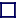### GUAGE BOSONS

Guage Bosons are integral-spin particles that carry the forces. There are four sets for the four known forces: electromagnetic force, weak force, strong force and gravitational force.

The electromagnetic force is carried by photons, which are a spin-1 bosons. This is responsible for both magnetic and electric fields, and holds electrons in orbit around nuclei to make atoms. The rest mass of a photon is zero. The photon travels at the speed of light, indeed, it is light. The photon is electrically neutral and so cannot act as a source of electomagnetic fields. Thus photons cannot interact directly with one another, and will easily pass straight through each other or travel in opposite directions towards each other without interplay. The only way photons can interact is if they turn themselves into a virtual electron/anti-electron (e+/e-) pair first, and this requires an intense beam such as in high energy lasers.

The weak force is carried by 3 bosons, two charged (W+ and W-), and one neutral Z0. These bosons are responsible for mediating beta (and inverse beta) decay. They are all massive, hence beta decay is an improbable event. All have spin-1. These bosons, being very heavy, must travel less than the speed of light. The mass of the W particles is 80.6GeV, that of the Z0 particle 91.177GeV.

The strong force is carried by eight coloured gluons, all spin-1 particles, all mass-less at rest. This force is responsible for binding quarks together, either as quark/anti-quark pair (for mesons) or as three quark triplets within baryons (hadrons) such as the proton or the neutron. These bosons have mass when bound together within nuclei, and hence travel at less than the speed of light therein. Gluons are non-Abelian, and thus can interact with themselves, and may bind together to form 'glueballs'.

The gravitational force is carried by the graviton, a spin-2 boson. The graviton need not travel at the speed of light, but there is good evidence that it does travel at or very near to the speed of light. If gravity travelled slower than light, then the ultra high energy cosmic rays (1020 to 1021eV) that are travelling at very close to the speed of light would have been slowed down in traversing the Universe because they would radiate gravitational Cerenkov radiation. The fact that ultra-high energy cosmic rays exist rules out gravitons travelling much slower than light-speed.

Bosons with even spin (0 or 2) always attract; those with odd spin (1) can either attract or repel. Thus if anti-gravity exists, then this must be mediated by an odd-spin boson (the graviton is an even-spin boson [spin-2]- the gravity we know always attracts).

Two fermions (½-integral spin particles) can pair up (with opposite spins) to become a composite particle with integer-spin, in other words, a boson. This happens in superconductivity, where two electrons pair-up, and in mesons, where a quark and anti-quark pair-up. [In superconductors, however, it is theoretically possible for electrons to pair up, not with opposite spin, but with the same spin - but only in 2004 has one superconductor been found that does this].

All bosons obey Bose-Einstein statistics, whereby all particles can have exactly the same energy levels, they need not, but they can, and when they do the result is called a Bose-Einstein Condensate.